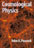© CAMBRIDGE UNIVERSITY PRESS 1999

### 3. THE ISOTROPIC UNIVERSE

3.1 The Robertson-Walker Metric

To the relativist, cosmology is the task of finding solutions to Einstein's field equations that are consistent with the large-scale matter distribution in the universe. Modern observational cosmology has demonstrated that the real universe is highly symmetric in its large-scale properties, but the evidence for this was not known at the time when Friedmann and Lemaître began their pioneering investigations. Just as Einstein aimed to write down the simplest possible relativistic generalization of the laws of gravity, so cosmological investigation began by considering the simplest possible mass distribution: one whose properties are homogeneous (constant density) and isotropic (the same in all directions).

ISOTROPY IMPLIES HOMOGENEITY At first sight, one might think that these two terms mean the same thing, but it is possible to construct universes that are homogeneous but anisotropic; the reverse, however, is not possible. Consider an observer who is surrounded by a matter distribution that is perceived to be isotropic; this means not only that the mass density is a function of radius only, but that there can be no preferred axis for other physical attributes such as the velocity field. This has an important consequence if we take the velocity strain tensor ðvi / ðxj and decompose it into symmetric and antisymmetric parts. The antisymmetric part corresponds to a rotation, so the velocity field can be written to first order in r as(3.1)

For isotropy, not only mustvanish, but all the principal values of the shear tensormust be equal. Thus, the only allowed velocity field on a local scale is expansion (or contraction) with velocity proportional to distance: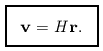(3.2)

Now, as was discovered by Slipher in the 1920s, we do in fact see such an isotropic recession about us; what does it tell us about the universe? It is easy to produce an isotropic velocity field: just set off an explosion that scatters debris in all directions with a range of speeds v. If the amount of matter involved were too small for self-gravity to be important, then the distance travelled by a piece of debris would be just vt and an observer left in the centre would inevitably see an isotropic distribution of objects with a recessional velocity proportional to their distance. However, most scientists believe that it is not reasonable to adopt a cosmological model in which the universe is simply a joke played for the benefit of mankind. This attitude is encapsulated in the Copernican principle, which states that humans are not privileged observers. Although obviously false on a local scale (a point selected at random would be most unlikely to lie so close to a star), there is no reason why our existence should depend on special features of the large-scale appearance of the universe. It is therefore a reasonable supposition that, if the universe appears isotropic about our position, it would also appear isotropic to observers in other galaxies; the term ``isotropic'' is therefore often employed in cosmology as a shorthand for ``isotropic about all locations''.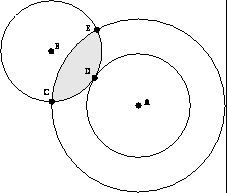Figure 3.1. Isotropy about two points A and B shows that the universe is homogeneous. From isotropy about B, the density is the same at each of C, D, E. By constructing spheres of different radii about A, the shaded zone is swept out and shown to be homogeneous. By using large enough shells, this argument extends to the entire universe.

This is a crucial shift in meaning, for the properties of such a universe are highly restricted. In fact, given only two points (A and B) from which conditions appear isotropic, we can prove that they must be homogeneous everywhere. Construct a sphere about A, on which the density has a constant value; considering the intersection of this sphere with similar ones centred on B shows that the density must be constant everywhere within the sphere, which can then be made as large as we desire (figure 3.1). Observationally, this may seem rather abstract, since it is not easy to verify isotropy from another's viewpoint. However, a universe that is isotropic about one point and which is homogeneous has to be isotropic in the general sense. Consider the expansion: since. v must be a (homogeneous) constant, vr; the expansion is therefore isotropic about every point, since v1 - v2r1 - r2. We now see how it is possible to imagine universes that are homogeneous but anisotropic. For example, a model that expands at different rates along three principal axes would preserve a homogeneous density, but the distance-redshift relation could be a function of direction on the sky.

Having chosen a model mass distribution, the next step is to solve the field equations to find the corresponding metric. Since our model is a particularly symmetric one, it is perhaps not too surprising that many of the features of the metric can be deduced from symmetry alone - and indeed will apply even if Einstein's equations are replaced by something more complicated. These general arguments were put forward independently by H.P. Robertson and A.G. Walker in 1936.

COSMOLOGICAL TIME The first point to note is that something suspiciously like a universal time exists in an isotropic universe. Consider a set of observers in different locations, all of whom are at rest with respect to the matter in their vicinity (these characters are usually termed fundamental observers). We can envisage them as each sitting on a different galaxy, and so receding from each other with the general expansion (although real galaxies have in addition random velocities of order 100 km s-1 and so are not strictly fundamental observers). We can define a global time coordinate t, which is the time measured by the clocks of these observers - i.e. t is the proper time measured by an observer at rest with respect to the local matter distribution. The coordinate is useful globally rather than locally because the clocks can be synchronized by the exchange of light signals between observers, who agree to set their clocks to a standard time when e.g. the universal homogeneous density reaches some given value. Using this time coordinate plus isotropy, we already have enough information to conclude that the metric must take the following form: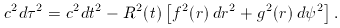(3.3)

Here, we have used the equivalence principle to say that the proper time interval between two distant events would look locally like special relativity to a fundamental observer on the spot: for them, c2 d2 = c2 dt2 - dx2 - dy2 - dz2. Since we use the same time coordinate as they do, our only difficulty is in the spatial part of the metric: relating their dx etc. to spatial coordinates centred on us.

Because of spherical symmetry, the spatial part of the metric can be decomposed into a radial and a transverse part (in spherical polars, d2 = d2 + sin2d2). Distances have been decomposed into a product of a time-dependent scale factor R (t) and a time-independent comoving coordinate r. The functions f and g are arbitrary; however, we can choose our radial coordinate such that either f = 1 or g = r2, to make things look as much like Euclidean space as possible. Furthermore, we can determine the form of the remaining function from symmetry arguments.

To get some feeling for the general answer, it should help to think first about a simpler case: the metric on the surface of a sphere. A balloon being inflated is a common popular analogy for the expanding universe, and it will serve as a two-dimensional example of a space of constant curvature. If we call the polar angle in spherical polars r instead of the more usual, then the element of length on the surface of a sphere of radius R is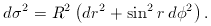(3.4)

It is possible to convert this to the metric for a 2-space of constant negative curvature by the device of considering an imaginary radius of curvature, R -> iR. If we simultaneously let r -> ir, we obtain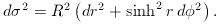(3.5)

These two forms can be combined by defining a new radial coordinate that makes the transverse part of the metric look Euclidean: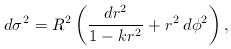(3.6)

where k = +1 for positive curvature and k = -1 for negative curvature. This argument is hardly a proof that spaces of constant negative curvature exist and can be justified only by a fuller treatment. However, it does give a feeling for the meaning of the final result. Indeed, the equation we have just written is in fact the exact form of the spatial part of the Robertson-Walker metric, which applies in any number of dimensions. The fact that we can obtain the correct answer using a 2-space is not so surprising in retrospect, because of isotropy: we only need consider the non-Euclidean nature of the geometry in a plane to determine the functions f and g.

It is clear that this metric nicely incorporates the idea of a uniformly expanding model with no centre. For small separations, where space is Euclidean, we have a simple scaling-up of vector separations: x (t)R (t) x (t0). The same law applies irrespective of the origin we choose: x1 (t) - x2 (t)R (t) [x1 (t0) - x2 (t0)]. Every fundamental observer with a sufficiently large ego will conclude that the universe is centred on them.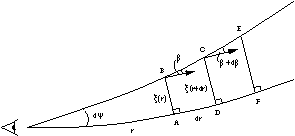Figure 3.2. The geometry of two light rays in a curved space. The angle between them is dand the transverse separation is. The test for curvature is when a vector that makes an anglewith one of the rays is parallel-transported around the loop ABCD; if the angle changes to+ d, then dis a measure of the degree of curvature.

AN INFORMAL DERIVATION There exists an illuminating half-way house between the above hand-waving and a totally formal approach. This is an argument due to P.A.G. Scheuer (given in Longair 1986). Consider the geometry shown in figure 3.2:is the comoving separation between two radial light rays and the radial increment of comoving distance is dr, i.e. we are working with f = 1 and trying to find, which is equal to g (r) d. Consider also a vector that makes an anglewith one of the light rays. The effect of non-Euclidean geometry will be to cause this angle to change by some amounton parallel transport around the loop. For our present purposes the crucial step in the argument is to see that isotropy and homogeneity require that this change must be proportional to the area of the loop. For example, going around the loop ABCD twice clearly produces twice theobtained from one circuit, but from homogeneity this should be the same as going round two identical loops, ABCD followed by DCEF (the multiple traversing of the line CD has no effect). Similarly, one can argue that the effect of any loop should be the sum of the effects of the sub-loops into which it may be divided. Given this, it is straightforward to derive, since= ð/ ðr;area therefore becomes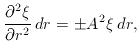(3.7)

where the constant of proportionality could have either sign. Apart from the Euclidean case, A = 0, there are thus two possible geometries: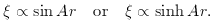(3.8)

If we impose the boundary condition-> r das r -> 0, then the constants of proportionality may be found. We end up with the same metric as we obtained from the 2-sphere. It is possible to give derivations of considerably greater rigour (consult Weinberg 1972 or Misner, Thorne & Wheeler 1973 for examples). However, this will suffice here.

The conclusion of this argument is that an isotropic universe has the same form for the comoving spatial part of its metric as the surface of a sphere. This is no accident, since it it possible to define the equivalent of a sphere in higher numbers of dimensions, and the form of the metric is always the same. For example, a 3-sphere embedded in four-dimensional Euclidean space would be defined as the coordinate relation x2 + y2 + z2 + w2 = R2. Now define the equivalent of spherical polars and write w = R cos, z = R sincos, y = R sinsincos, x = R sinsinsin, where,andare three arbitrary angles. Differentiating with respect to the angles gives a four-dimensional vector (dx, dy, dz, dw), and it is a straightforward exercise to show that the squared length of this vector is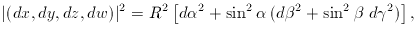(3.9)

which is the Robertson-Walker metric for the case of positive spatial curvature. This k = +1 metric describes a closed universe, in which a traveller who sets off along a trajectory of fixedandwill eventually return to their starting point (when= 2). In this respect, the positively curved 3D universe is identical to the case of the surface of a sphere: it is finite, but unbounded. By contrast, the k = -1 metric describes an open universe of infinite extent; as before, changing to negative spatial curvature replaces sinwith sinh, andcan be made as large as we please without returning to the starting point. The k = 0 model describes a flat universe, which is also infinite in extent. This can be thought of as a limit of either of the k = ± 1 cases, where the curvature scale R tends to infinity.

NOTATION AND CONVENTIONS The Robertson-Walker metric (which we shall often write in the shorthand RW metric) may be written in a number of different ways. The most compact forms are those where the comoving coordinates are dimensionless. Define the very useful function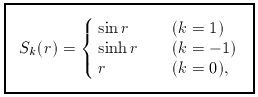(3.10)

and its cosine-like analogue, which will be useful later,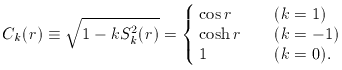(3.11)

The metric can now be written in the preferred form that we shall use throughout: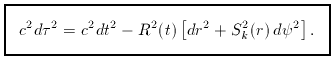(3.12)

The most common alternative is to use a different definition of comoving distance, Sk (r) -> r, so that the metric becomes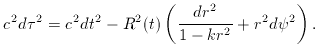(3.13)

There should of course be two different symbols for the different comoving radii, but each is often called r in the literature, so we have to learn to live with this ambiguity; the presence of terms like Sk (r) or 1 - kr2 will usually indicate which convention is being used. Alternatively, one can make the scale factor dimensionless, defining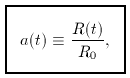(3.14)

so that a = 1 at the present. In this case, the metric becomes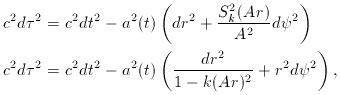(3.15)

where A is the constant of proportionality from Scheuer's argument, which is equal to R0, the current value of R (t). We shall generally use the metric in the form with dimensionless comoving distances. However, it is important to note that cosmologists tend to use the term ``distance'' as meaning comoving distance unless otherwise specified, usually in units of Mpc: this means that one generally deals with the combination R0 r.

Other forms of the metric are encountered less commonly; in particular, the rarely used isotropic form is(3.16)

There is, however, one other modification of some importance; this is to define the conformal time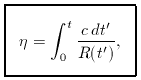(3.17)

which allows a factor of R2 to be taken out of the metric. This is a special case of a conformal transformation in general relativity, such transformations correspond to gµ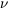-> fgµ, where f is some arbitrary spacetime function. The universe with k = 0, although certainly possessing spacetime curvature, is obviously directly related to Minkowski spacetime via a conformal transformation and so tends to be loosely known as the ``flat'' model. In fact, the Robertson-Walker metric turns out to be conformally flat for all values of k - i.e. a coordinate transformation can always be found that casts the metric in the form of the Minkowski metric multiplied by some spacetime function.

COMOVING SEPARATION Having erected a comoving coordinate system about a given observer, we can calculate the comoving separation of two events (or astronomical ``sources'') that lie close to each other. How does this generalize to the case when dr and dare not small? The analogy with a 2-sphere is invaluable in this case, as the answer is obviously just the spherical cosine rule (appropriately generalized for positive and negative curvature):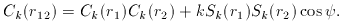(3.18)

This can be recast in terms of the more usual Sk(r) via Sk2 = (1 - Ck2) / k: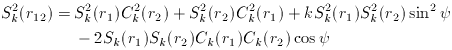(3.19)

For a flat universe (k = 0), this goes over to the cosine rule, as expected. To see how complicated a non-intuitive derivation of this result can become, see Osmer (1981).

THE REDSHIFT It is time to re-establish contact with the expanding universe in terms of Hubble's law: v = Hr. Our discussion so far has been in terms of comoving coordinates, which are time independent. At small separations, where things are Euclidean, it is easy enough to see what happens: the proper separation of two fundamental observers is just R (t) dr, so that we obtain Hubble's law with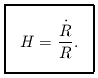(3.20)

At large separations where spatial curvature becomes important, the concept of radial velocity becomes a little more slippery - but in any case how could one measure it directly in practice? At small separations, the recessional velocity gives the Doppler shift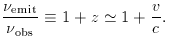(3.21)

This defines the redshift z in terms of the shift of spectral lines. What is the equivalent of this relation at larger distances? Since photons travel on null geodesics of zero proper time, we see directly from the metric that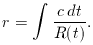(3.22)

The comoving distance is constant, whereas the domain of integration in time extends from temit to tobs; these are the times of emission and reception of a photon. Photons that are emitted at later times will be received at later times, but these changes in temit and tobs cannot alter the integral, since r is a comoving quantity. This requires the condition dtemit / dtobs= R (temit) / R(tobs), which means that events on distant galaxies time-dilate according to how much the universe has expanded since the photons we see now were emitted. Clearly (think of events separated by one period), this dilation also applies to frequency, and we therefore get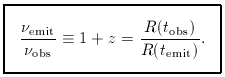(3.23)

In terms of the normalized scale factor a (t) we have simply a (t) = (1 + z)-1. Photon wavelengths therefore stretch with the universe, as is intuitively reasonable; see figure 3.3.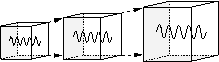Figure 3.3. Suppose we trap some radiation inside a box with silvered sides, that expands with the universe. At least for an isotropic radiation field, the photons trapped in the box are statistically equivalent to any that would pass into this space from outside. Since the walls expand at v << c for a small box, it is easily shown that the Doppler shift maintains an adiabatic invariant, which is the ratio of wavelength to box side, and so radiation wavelengths increase as the universe expands. This argument also applies to quantum-mechanical standing waves: momentum declines as a (t)-1.

This is the only correct interpretation of the redshift at large distances; it is common but misleading to convert a large redshift to a recession velocity using the special-relativistic formula 1 + z = [(1 + v/c) / (1 - v/c)]1/2. Any such temptation should be avoided - it is only valid in a universe with zero matter density [problem 3.4].